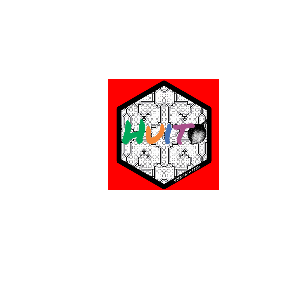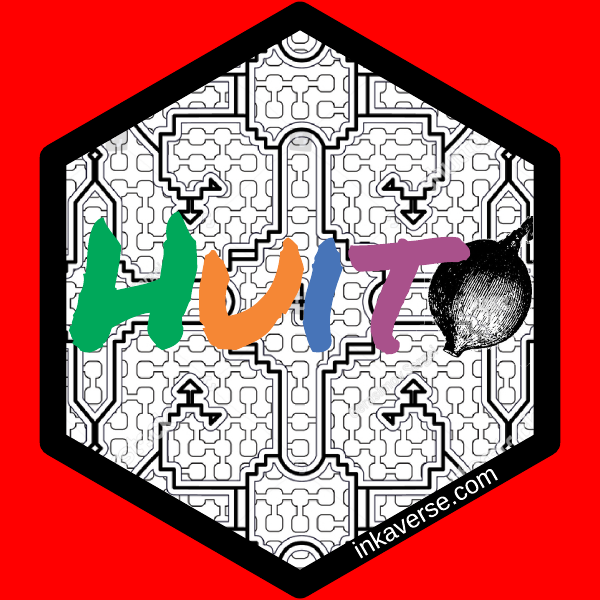# Stickers

library(huito)

font <- "Permanent Marker"

huito_fonts(font)

label <- label_layout(size = c(5.08, 5.08)
, border_color = NA
, border_width = 0
) %>%
include_image(value = "https://flavjack.github.io/huito/img/shipibo.png"
, size = c(7, 7)
, position = c(2.55, 2.52)
) %>%
include_text(value = "H", size = 45 , position = c(1.15, 2.7), color = "#00a85a", font) %>%
include_text(value = "u", size = 45 , position = c(2.07, 2.7), color = "#f58735", font) %>%
include_text(value = "i", size = 45 , position = c(2.73, 2.7), color = "#4774b8", font) %>%
include_image(value = "https://flavjack.github.io/huito/img/huito_fruit.png"
, size = c(1.3, 1.3)
, position = c(4.06, 2.6)
) %>%
include_text(value = "t", size = 45 , position = c(3.33, 2.7), color = "#a9518b", font) %>%
include_shape(size = 4.1
, border_width = 3
, border_color = "black"
, margin = -0.8
, position = c(2.54, 2.54)
, panel_color = "red"
, panel_size = 5.08
) %>%
include_text(value = "inkaverse.com"
, size = 6
, position = c(3.6, 0.75)
, angle = 30
, color = "white") 

Select at panel_color() different from the colors in your stickers background.

## Preview mode

label %>%
label_print(mode = "preview")## Complete mode

sticker <- label %>%
label_print(filename = "huito"
, margin = 0
, paper = c(5.5, 5.5)
, viewer = T
, smpres = 300
, mode = "complete"
)